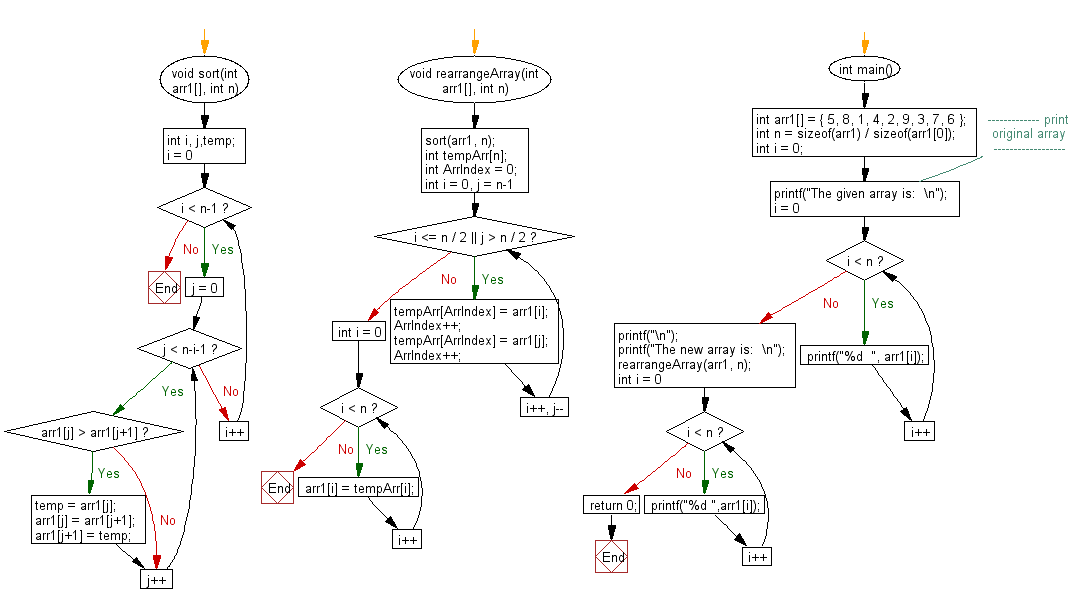﻿ C exercises: Rearrange an array in such an order that– smallest, largest, 2nd smallest, 2nd largest and on - w3resource# C Exercises: Rearrange an array in such an order that– smallest, largest, 2nd smallest, 2nd largest and on

## C Array: Exercise-102 with Solution

Write a program in C to rearrange an array in such an order that– smallest, largest, 2nd smallest, 2nd largest and on.

Sample Solution:

C Code:

``````#include<stdio.h>
void sort(int arr1[], int n)
{
int i, j,temp;
for (i = 0; i < n-1; i++)
{
for (j = 0; j < n-i-1; j++)
{
if (arr1[j] > arr1[j+1])
{
temp = arr1[j];
arr1[j] = arr1[j+1];
arr1[j+1] = temp;
}
}
}
return ;
}

void rearrangeArray(int arr1[], int n)
{
sort(arr1, n);
int tempArr[n];
int ArrIndex = 0;
for (int i = 0, j = n-1; i <= n / 2 || j > n / 2; i++, j--)
{
tempArr[ArrIndex] = arr1[i];
ArrIndex++;
tempArr[ArrIndex] = arr1[j];
ArrIndex++;
}
for (int i = 0; i < n; i++)
{arr1[i] = tempArr[i];}
}

int main()
{
int arr1[] = { 5, 8, 1, 4, 2, 9, 3, 7, 6 };
int n = sizeof(arr1) / sizeof(arr1);
int i = 0;
//------------- print original array ------------------
printf("The given array is:  \n");
for(i = 0; i < n; i++)
{
printf("%d  ", arr1[i]);
}
printf("\n");
//-----------------------------------------------------------
printf("The new array is:  \n");
rearrangeArray(arr1, n);
for (int i = 0; i < n; i++)
printf("%d ",arr1[i]);
return 0;
}
```
```

Sample Output:

```The given array is:
5  8  1  4  2  9  3  7  6
The new array is:
1 9 2 8 3 7 4 6 5
```

Pictorial Presentation:Flowchart:C Programming Code Editor:

Improve this sample solution and post your code through Disqus.

﻿